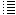11.7 Confinement of positive ions and electrons with a static electric and magnetic fieldExperiments with the simulation program: exp. 11.7 till 11.9

Back to main page

 Experiment 11.7 32 positive charges of each 8,3E-7 C (300 kV) are placed in the upper horizontal side and in the lower horizontal side, see screenshot and video. In the program dt=1.59E-10 A (solitary) sphere with diameter 5 cm and a voltage of +300 kV (to infinity) has a charge of: qi= 3E5/(9E9)* 0.025 = 8,3E-07 Coulomb. Bfield: 1,5 tesla (constant) ve=3E6 m/s {=max. initial speed} 20 H+ ions were generated: hydrogen[i].vx:=0 + (-0.5 + random)*ve; hydrogen[i].vy:=0+( - 0.5 + random)*ve; hydrogen[i].vz:=0 +( - 0.5 + random)*ve; hydrogen[i].x:=0.5+ ( - 0.5 + random) /5; {so they start not in exactly the same point} hydrogen[i].y:=0.5+ ( - 0.5 + random) /5; hydrogen[i].z:=0.5 + ( - 0.5 + random) /10; 20 B+ ions were generated: boron[i].vx:=0 + ( - 0.5 + random)*ve; boron[i].vy:=0 + ( - 0.5 + random)*ve; boron[i].vz:=0 + ( - 0.5 + random)*ve; boron[i].x:=0.5 + ( - 0.5 + random) /9; boron[i].y:=0.5 + ( - 0.5 + random) /5; boron[i].z:= 0.5+ ( - 0.5 + random) /10; After 1,07E-4 s the positive ions stay confined in the simulation space. The total energy of the ions (kinetic + potential) stayed contant = 2,26 ±0,01E-12 J, which is an indication (but not proof) that the simulation is correct (non-relativistic). Experiment 11.8 32 positive charges of each 5.56E-07 coulomb  (200 kV) are placed in the upper horizontal side and in the lower horizontal side, see screenshot and video. In the program dt=1.59E-10 A (solitary) sphere with diameter 5 cm and a voltage of +200 kV (to infinity) has a charge of: qi=  5.56E-07 Coulomb. Bfield: 1 tesla (constant) massa hydrogen ion  mp=2*1.6726E-27 = massa deuterium ion ve=3E6 m/s {=max. initial speed} 30 D ions were generated:  (double mass H+) vx:=0 + (-0.5 + random)*ve; vy:=0+( - 0.5 + random)*ve; vz:=0 +( - 0.5 + random)*ve; x:=0.5+ ( - 0.5 + random) /5; {so they start not in exactly the same point} y:=0.5+ ( - 0.5 + random) /5; z:=0.5 + ( - 0.5 + random) /10; no B+ ions were generated: After 7,5E-5 s the positive deuterium ions stayed confined in the simulation space. The total energy of the ions (kinetic + potential) stayed contant = 1,68  E-12 J, which is an indication (but not proof) that the simulation is correct (non-relativistic). Experiment 11.9 32 positive charges of each 2.78E-07 coulomb  (100 kV) are placed in the upper horizontal side and in the lower horizontal side, see screenshot and video. In the program dt=1.59E-10 A (solitary) sphere with diameter 5 cm and a voltage of +100 kV (to infinity) has a charge of: qi=  2.78E-07 Coulomb. Bfield: 0.7 tesla (constant) massa hydrogen ion  mp=2*1.6726E-27 = massa deuterium ion ve=3E6 m/s {=max. initial speed} 30 D ions were generated:  (double mass H+) vx:=0 + (-0.5 + random)*ve; vy:=0+( - 0.5 + random)*ve; vz:=0 +( - 0.5 + random)*ve; x:=0.5+ ( - 0.5 + random) /5; {so they start not in exactly the same point} y:=0.5+ ( - 0.5 + random) /5; z:=0.5 + ( - 0.5 + random) /10; no B+ ions were generated: After 9.4 E-5 s the positive deuterium ions stayed confined in the simulation space. The total energy of the ions (kinetic + potential) stayed contant = 1,08  E-12 J, which is an indication (but not proof) that the simulation is correct (non-relativistic). The same experiment, but with 100 D+ ions and 90 kV. The same result, see screenshot:

Back to the main page